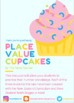# Place Value Cupcakes - Number Knowledge and Place Value PracticeSubject
Resource Type
File Type

PDF

(78 KB)
Product Rating
Standards
• Product Description
• StandardsNEW

Your students will work on their number knowledge and place value in this cute activity!

They will decorate their cupcakes with base

There are three levels of difficulty in this task, and a break down of the activity.

Level One: Three digit numbers (aligned to Stage 4 of the NZ Curriculum)

• Total value (number form)
• Identify the number in the hundreds, tens and ones OR how many hundreds, tens and ones are in each number.
• One more, one less
• Ten more, ten less
• Expanded form
• Written form

Level Two: Four digit numbers (aligned to Stage 5 of the NZ Curriculum)

• Total value (number form)
• Identify the number in the thousands, hundreds, tens and ones OR how many thousands, hundreds, tens and ones are in each number.
• Ten more, Ten less
• One hundred more, one hundred less
• Expanded form
• Written form

Level Three: Tens to Hundredths (aligned to Stage 6/7 of the NZ Curriculum

• Total value (number form)
• Identify the number in the tens, ones, tenths, hundredths OR how many tens, ones, tenths and hundredths are in each number.
• One hundredth more, one hundredth less
• One tenth more, one tenth less
• Ten more, ten less
• Written form

You can use this activity as a tool in a teacher directed lesson, or as a follow up activity - which can be done in pairs or individually.

Read and write decimals to thousandths using base-ten numerals, number names, and expanded form, e.g., 347.392 = 3 × 100 + 4 × 10 + 7 × 1 + 3 × (1/10) + 9 × (1/100) + 2 × (1/1000).
Read, write, and compare decimals to thousandths.
Read and write multi-digit whole numbers using base-ten numerals, number names, and expanded form. Compare two multi-digit numbers based on meanings of the digits in each place, using >, =, and < symbols to record the results of comparisons.
Total Pages
N/A
N/A
Teaching Duration
N/A
Report this Resource to TpT
Reported resources will be reviewed by our team. Report this resource to let us know if this resource violates TpT’s content guidelines.